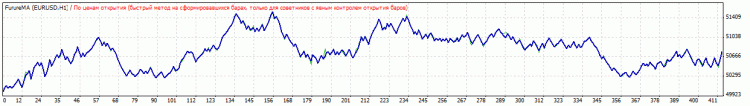# Future___ – expert for MetaTrader 4

• A+

Attempt to create a grid encouraged me to do this.

Take N+M bars on history interval. Write the indicator signals among N bars, and the result among M bars.

This results in a file named [sequence of indicator signals from N bars].csv.

The file contains the subsequent history results.

For example, write the following to a small block:

```      int history ;
for (int i=analiz_bars+poisk_fractals;i>poisk_fractals;i--)
{
history [i] = NormalizeDouble((tocnost*iMACD(NULL,0,Per_MACD/4,Per_MACD,9,PRICE_CLOSE,MODE_MAIN, i)/(100*Point)),0);
}```

It outputs an array which is further used here:

```      string posledovatelnost = shethik +"_";
for (i=analiz_bars+poisk_fractals;i>poisk_fractals;i--)
{
posledovatelnost =posledovatelnost+history [i]+"_";
}
posledovatelnost = posledovatelnost +".csv";```

Then save the file with the name [posledovatelnost].

Write the number of calls (to determine the statistical weight) and results of the following block to that file:

```      double MaxHighPik = High [poisk_fractals]; //Start comparing the bars
double MaxLowPik = Low [poisk_fractals];
for (i=poisk_fractals; i>=1; i--)
{
if (MaxHighPik < High [i])
{
MaxHighPik = High [i];
}
if (MaxLowPik > Low [i])
{
MaxLowPik = Low [i];
}
}

double Fractal_Up = (MaxHighPik - Open [poisk_fractals])/Point;
double Fractal_Down = (Open [poisk_fractals]-MaxLowPik)/Point;```

The movement direction of the following N bars and the reached maximums are determined here. The results are written as averaged with the ability to assign the more recent data an increased or decreased statistical weight using the zabyvaemost variable. The indicator values are additionally averaged by the tocnost variable. With minimal averaging only the direction +1 or -1 is given.

But. Despite the wonderful looks the results are not impressive. Two expert advisors are provided as example. Profitability rarely rises above 1.1

Example of using the MA:If anyone manages to make a decent EA, please contact me.